The graph which represents the variation of mean kinetic energy of molecules with temperature $t°C$ is ?

(1)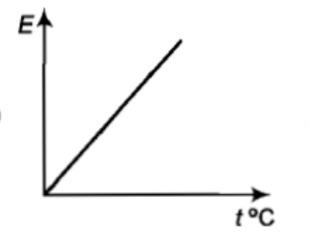(2)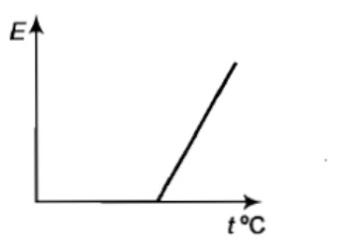(3)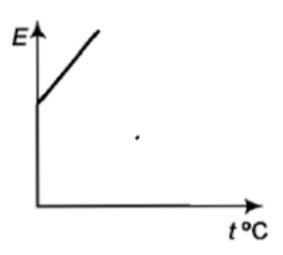(4)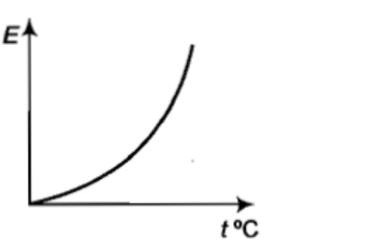Concept Questions :-

Kinetic energy of gas
High Yielding Test Series + Question Bank - NEET 2020

Difficulty Level:

${V}_{rms},{V}_{av},{V}_{mp}$ are root mean square, average and most probable speeds of molecules of a gas obeying Maxwellian velocity distribution. Which of the following statements is correct?

1. ${V}_{rms}<{V}_{av}<{V}_{mp}$

2. ${V}_{rms}>{V}_{av}>{V}_{mp}$

3.

4. ${V}_{avg}>{V}_{rms}<{V}_{mp}$

Concept Questions :-

Types of velocity
High Yielding Test Series + Question Bank - NEET 2020

Difficulty Level:

Gas is found to obey the law ${P}^{2}V$ = constant. The initial temperature and volume are ${T}_{0}$ and ${V}_{0}$. If the gas expands to a volume $3{V}_{0}$, its final temperature becomes:

(a) $\frac{{T}_{0}}{3}$           (b) $\frac{{T}_{0}}{\sqrt{3}}$

(c) $3{T}_{0}$           (d) None of these

Concept Questions :-

Ideal gas
High Yielding Test Series + Question Bank - NEET 2020

Difficulty Level:

For a gas, the r.m.s speed at 800 K is:

(1) Four times the value at 200 K

(2) Half the value at 200 K

(3) Twice the value at 200 K

(4) Same as at 200 K

Concept Questions :-

Types of velocity
High Yielding Test Series + Question Bank - NEET 2020

Difficulty Level:

The average kinetic energy of a helium atom at $30°C$ is:                                                                                           [MP PMT 2004]

(1) Less than 1 eV

(2) A few KeV

(3) 50-60 eV

(4) 13.6 eV

Concept Questions :-

Kinetic energy of gas
High Yielding Test Series + Question Bank - NEET 2020

Difficulty Level:

The mean translational kinetic energy of a perfect gas molecule at the temperature T Kelvin is:

(1) $\frac{1}{2}kT$         (2) kT             (3) $\frac{3}{2}kT$            (4) 2 kT

Concept Questions :-

Kinetic energy of gas
High Yielding Test Series + Question Bank - NEET 2020

Difficulty Level:

Choose the correct option related to the specific heat at constant volume ${C}_{V}$ -

(1) ${C}_{V}=\frac{1}{2}fR$              (2) ${C}_{V}=\frac{R}{\gamma -1}$

(3) ${C}_{V}=\frac{{C}_{P}}{\gamma }$                (4) All of these

Concept Questions :-

Specific heat
High Yielding Test Series + Question Bank - NEET 2020

Difficulty Level:

The curve between absolute temperature and ${{v}_{rms}}^{2}$ is:

(a)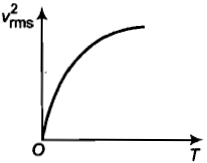(b)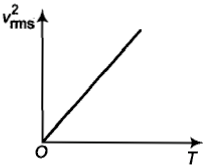(c)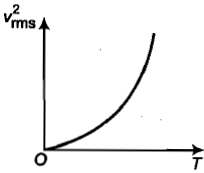(d)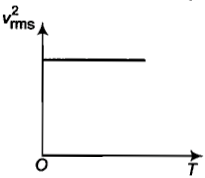Concept Questions :-

Types of velocity
High Yielding Test Series + Question Bank - NEET 2020

Difficulty Level:

Five molecules of a gas have speeds, 1, 2, 3, 4 and 5 km${s}^{-1}$.  The value of the root mean square speed of the gas molecules is:

(1) 3 km${s}^{-1}$             (2) $\sqrt{11}$ km${s}^{-1}$

(3) $\frac{1}{2}\sqrt{\mathrm{\pi }}$ km${s}^{-1}$    (4) 3.5 km${s}^{-1}$

Concept Questions :-

Types of velocity
High Yielding Test Series + Question Bank - NEET 2020

Difficulty Level:

The average velocity of an ideal gas molecule is:                                                                                                                   [OJEE 2010]

(1) proportional to $\sqrt{T}$

(2) proportional to ${T}^{2}$

(3) proportional to ${T}^{3}$

(4) zero

Concept Questions :-

Types of velocity